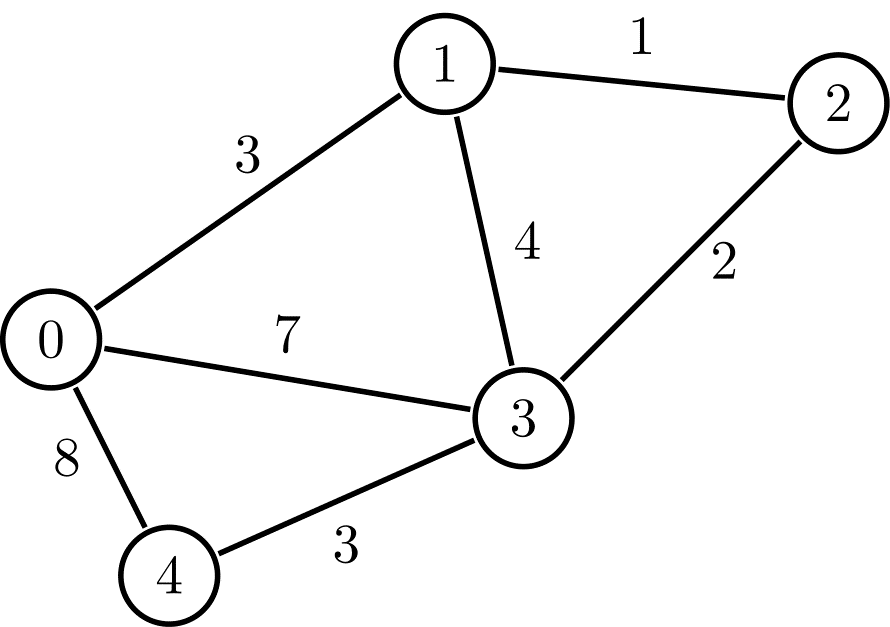Problem title
Difficulty
Avg time to solve

Repeat Triangle
Easy
15 mins
MegaPrime Numbers
Easy
15 mins
Mirror image of triangle
Easy
10 mins
Maximum Difference
Moderate
30 mins
Magician and Chocolates
Easy
15 mins
Possible Balanced Strings
Moderate
15 mins
Maximum value node
Easy
15 mins
Maximum 0-1 Distance
Hard
10 mins
Next Higher Node
Easy
15 mins
Path Queries
Moderate
25 mins2

Difficulty: EASY
Avg. time to solve
15 min
Success Rate
85%

Problem Statement

#### You live in the country with ‘V’ cities that have ‘E’ roads. You are in city ‘S’ with a car having ‘P’ amount of petrol in it. Roads are bidirectional and consume your petrol. Each road has a description ‘X’, ‘Y’ and ‘Z’, which means city ‘X’ and ‘Y’ have a road between them which consumes ‘Z’ amount of petrol. You want to visit the city ‘D’. Your task is to check if it's possible to visit ‘D’ from ‘S’ using ‘P’ amount of petrol.

##### For Example:
``````In the given graph, if we want to reach from node 1 to node 4 with ‘P’ = 10, then our answer is ‘Yes’ because there exists path 1 -> 3 -> 4, which takes 7 amount of petrol, which is less than the given value of ‘P’ so it is possible and hence, the answer is ‘Yes’.
``````##### Input Format:
``````The first line of the input contains a single integer, 'T,’ denoting the number of test cases.

The first line of each test case contains three space-separated integers, ‘V’, ‘E’, and ’P’, denoting the number of vertices, the number of edges in the graph, and the amount of petrol in the car.

The next ‘E’ lines of each test case contain three space-separated integers, ‘X’, ‘Y’ and ‘Z’, denoting the vertices which are connected with a bidirectional edge, and the weight of the edge.

The last line of each test case has two space-separated integers, ‘S’ and ’D’, representing the source and destination vertices.
``````
##### Output Format:
``````For each test case, print “Yes” if there exists a path from vertex 'S’ to 'D’ which consumes less than or equal to the given amount of petrol ‘P’. Otherwise, print “No” (without quotes).

Print the output of each test case in a separate line.
``````
##### Note:
``````You do not need to print anything, it has already been taken care of. Just implement the given function.
``````
##### Constraints :
``````1 <= T <= 10
2 <= V <= 2000
0 <= E <= (V*(V-1)) / 2
0 <= X,Y < V
1 <= Z <= 10 ^ 6
1 <= P <= 10 ^ 9
0 <= S,D < V

Time Limit: 1 sec
``````
##### Sample Input 1:
``````2
4 3 4
0 1 1
1 2 1
2 3 1
0 3
4 5 3
0 1 1
0 2 1
0 3 4
1 2 2
2 3 2
0 3
``````
##### Sample Output 1:
``````Yes
Yes
``````
##### Explanation:
``````In test case 1:
there are 4 vertices and 3 edges, and there is a path between 0 and 3 which is 0 -> 1 -> 2 -> 3. The path consumes 3 amount of petrol, which is less than the given ‘P’. Hence, the answer is ‘Yes’.

In test case 2:
there are 4 vertices and 5 edges, and there is a path between 0 and 3 which is 0 -> 2 -> 3.  The path consumes 3 amount of petrol, which is equal to the given ‘P’. Hence, the answer is ‘Yes’.
``````
##### Sample Input 2:
``````1
3 2 1
0 1 3
1 2 4
0 2
``````
##### Sample Output 2:
``````No
``````Console